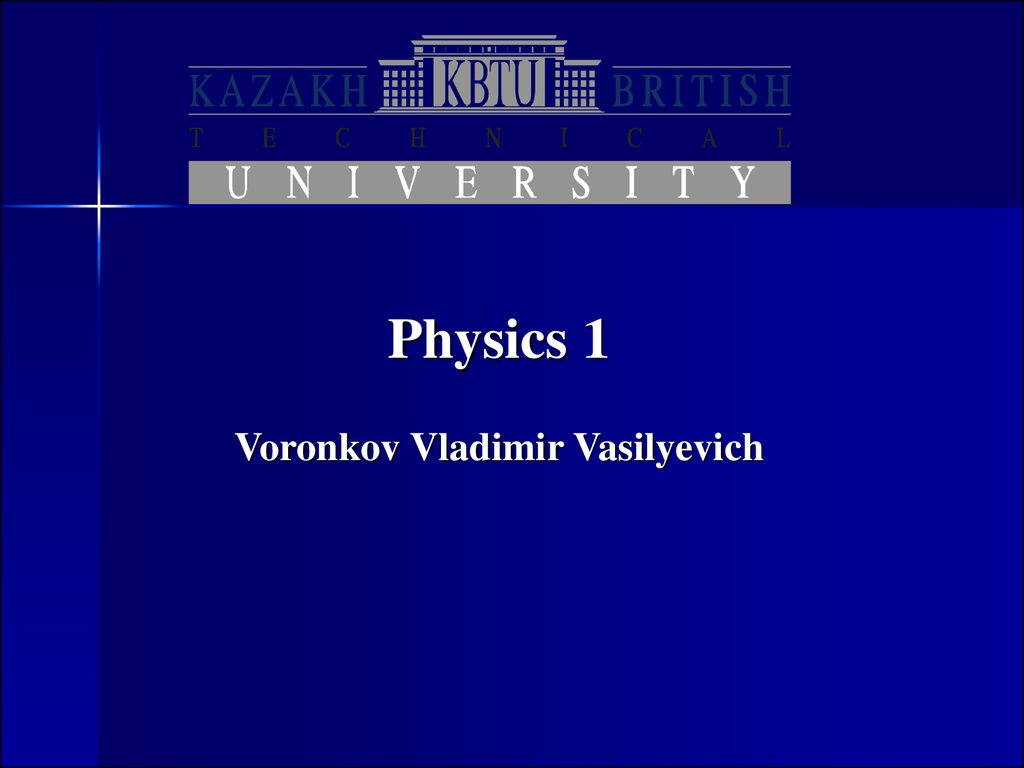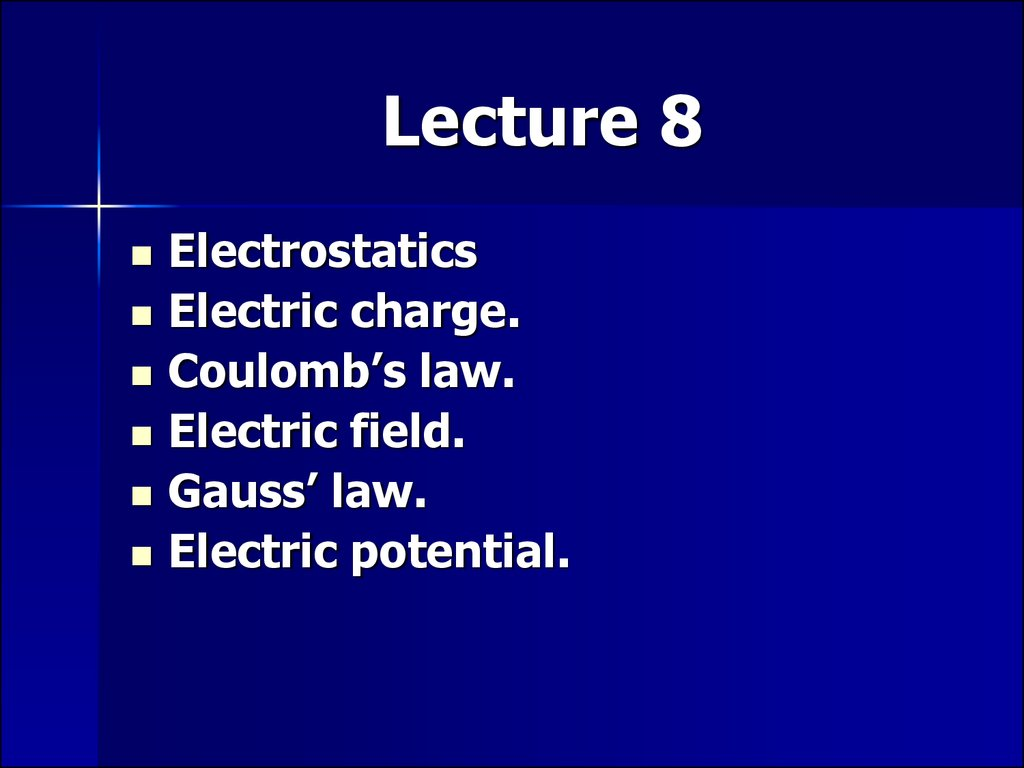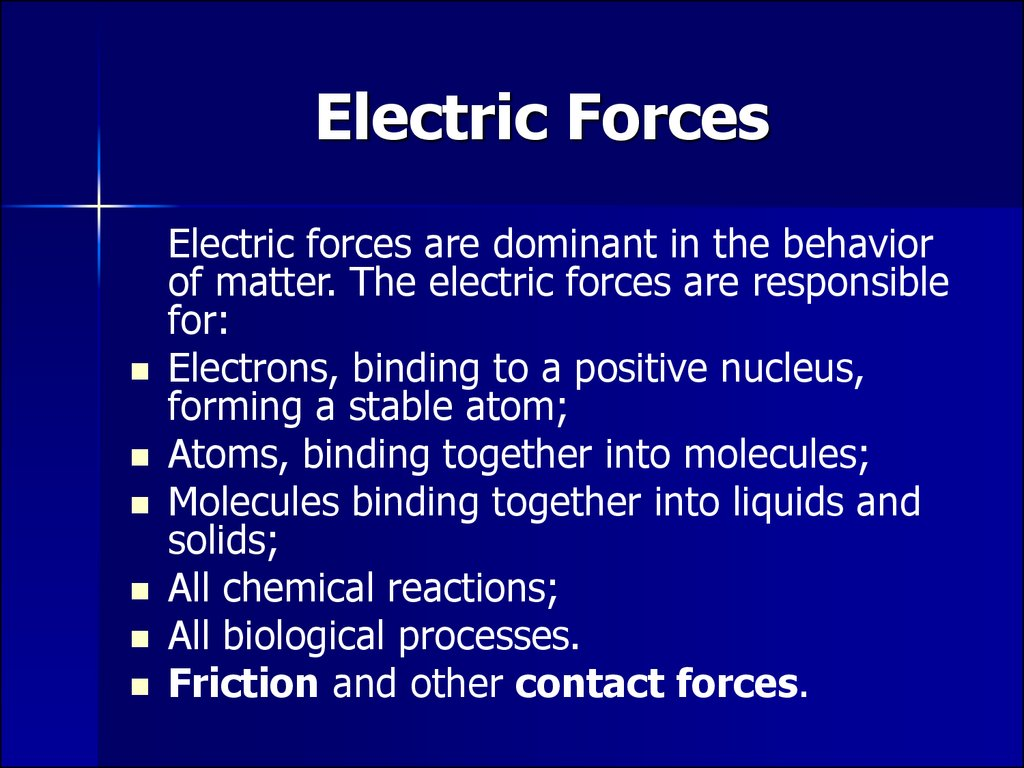# Electric Forces

Physics 1

## 2. Lecture 8

Electrostatics
Electric charge.
Coulomb’s law.
Electric field.
Gauss’ law.
Electric potential.

## 3. Electric Forces

Electric forces are dominant in the behavior
of matter. The electric forces are responsible
for:
Electrons, binding to a positive nucleus,
forming a stable atom;
Atoms, binding together into molecules;
Molecules binding together into liquids and
solids;
All chemical reactions;
All biological processes.
Friction and other contact forces.

## 4. Electrostatics

Electrostatics is the science of
stationary charges.
There exists two types of charges –
positive and negative.
If an object has an excess of
electrons, it is negatively charged; if it
has a deficiency of electrons, it is
positively charged.
Like charges repel, and unlike charges
attract.

## 5. Charging by induction

(a)
(b)
(c)
(d)
(e)
We have a neutrally charged
conductor.
Negatively charged rod polarizes
the sphere. The charge in the rod
repels electrons to the opposite
side of the sphere.
Then we ground the sphere and
some part of electrons is repelled
into the Earth. There is induced
positive charge near the rod.
Then ground connection is
removed.
Eventually, we get positively
charged sphere.

## 6. The Law of Conservation of Charge

Charge of an isolated system is
conserved.
This law is a fundamental physical
law: net charge is the same
before and after any interaction.

## 7. Elementary charges

Elementary charges are electrons and protons. Usually
only electrons can be free and take part in electrical
processes.
Excess of electrons causes negative charge and
deficiency of electrons causes positive charge of a
body.

## 8. Coulomb’s law

From Coulomb’s experiments, we can
generalize the following properties of the
electric force between two stationary point
charges:
– is inversely proportional to the square of the
separation r between the particles and directed
along the line joining them;
– is proportional to the product of the charges q1
and q2 on the two particles;
– is attractive if the charges are of opposite sign
and repulsive if the charges have the same sign;
– is a conservative force.

## 9. Coulomb’s Law

• The magnitude of the electric force is
is the Coulomb
constant, it can be written in the following form:
• where
electric permittivity of free space.
is the

## 10.

In a vector form, the force exerted by charge q1 on q2 is:
Where

is a unit vector directed from q1 to q2.
(a) two similar charges repels
(b) two different charges attracts

## 11. Forces of Multiple Charges

Electrostatic force is a vector quantity, so in the case of
multiple charges the principle of superposition is
applicable:
The total force on charge q2 is the
vector sum of all forces:

## 12. Electric Field

In general: field forces can act through
space, producing an effect even when no
physical contact occurs between interacting
objects.
Charges gives rise to an electric field.
The electric field can be detected at any
particular point by a small test positive
charge qo and observing if it experiences a
force. Then the electric field vector is:
Note: force Fe and field E are not produced
by the test charge qo .

## 14. Electric Field Vector

The force exerted by q on the test charge q0 is:
Then dividing it by q0 we get the electric field
vector:
Electric field is created by a charge.
If a charge is positive then the electric field
vector is directed away from the source charge.
If a charge is negative then the electric field
vector is directed to the source charge.

## 15. Continuous Charge Distribution

• Volume charge density
• Surface charge density
• Linear charge density

## 16. Electric Field of a Uniformly Charged ring

• A ring of radius a carries a uniformly
distributed positive total charge Q. Let’s find
the electric field due to the ring along the
central axis perpendicular to the plane of the
ring.

## 17.

dE is the eld at point P on the
x axis due to an element of
charge dq. dE has two
perpendicular components:
EX and E .
of
Using the symmetry:
The perpendicular component
the field at P due to segment 1
is canceled by the perpendicular
component due to segment 2.
Thus the total E is directed
along x axis.

## 18.

The distance from a charge dq to point P:
Then the contribution of a charge
dq to electric field E at point P is:
All segments of the ring make the same contribution to the
eld at P because they are all equidistant from this point.
Thus, we can integrate to obtain the total eld at P:

## 19. Extreme Case Analysis

• So we found the electric field of a uniformly charged ring along
its symmetry axis at distance x from the centre of a ring:
ke is the Coulomb constant, a – the ring’s radius, Q – the charge
of the ring.
• Let’s analyze the obtained result for extreme cases:
1. If x=0, then E=0.
2. If x>>a, then we get the Coulomb formula for a point charge:
Q
E ke 2
r
• Look more examples of calculating electric field for continuous
charge distribution:
– in Serway p.721-723,
– Fishbane 642-647.

## 20. Gauss’ Law

The net flux of electric field through any
enclosed surface are equal to the net charge
inside that surface divided by permittivity of
free space.
Here E·dA is a scalar product of electric field
and differential of area vectors.

## 21. Electric Flux

DAi is a vector, which magnitude represents the
area of the i-th element of the surface and
direction is de ned to be perpendicular to the
surface element.
The variation in the electric eld over one
element of surface can be neglected if the
element is suf ciently small.
The electric ux through this element is

## 22.

• According to the Gauss’
theorem electric flux through
any surface S1, S2, S3 is the
same.
• Electric flux from a charge
located outside a surface
equals zero. The number of
lines entering the surface
equals the number leaving
the surface and the net
number equals zero.

## 23. Electric Potential Energy

For infinitesimal displacement ds the work
done by the electric field on the charge is
.
Then the change in the potential energy of
the charge-field system is
Thus for finite displacement from A to B the
change in potential energy is
This line integral is not path-dependant, as
the electric force is conservative.

## 24. Electric Potential

The electric potential at any point in
an electric field is
The potential difference DV=VB - VA
between two points A and B in an
electric field is defined as
q0 is a test charge.

## 25. Potential Properties

Just as with potential energy, only
differences in electric potential are
meaningful.
Electric potential is a scalar characteristic
of an electric eld, independent of any
charges that may be placed in the electric
field.
Electric potential energy depends on the
magnitude of the charge, interacting with
the field.

## 26. Units in SI

Charge
Electric potential
Electric field
Q
V
E
C (Coulomb)
J/C=V (volt)
N/C=V/m